### Infinity

In this last introductory quiz, we'll investigate some visual tools for exploring the infinitely small and, by using hyperbolic space instead of Euclidean, the infinitely large as well.

Even though there are only ever finitely many pixels at our disposal on the screen, these visualizations will be used to symbolically represent and build intuition for infinite sequences, sums, and geometric spaces.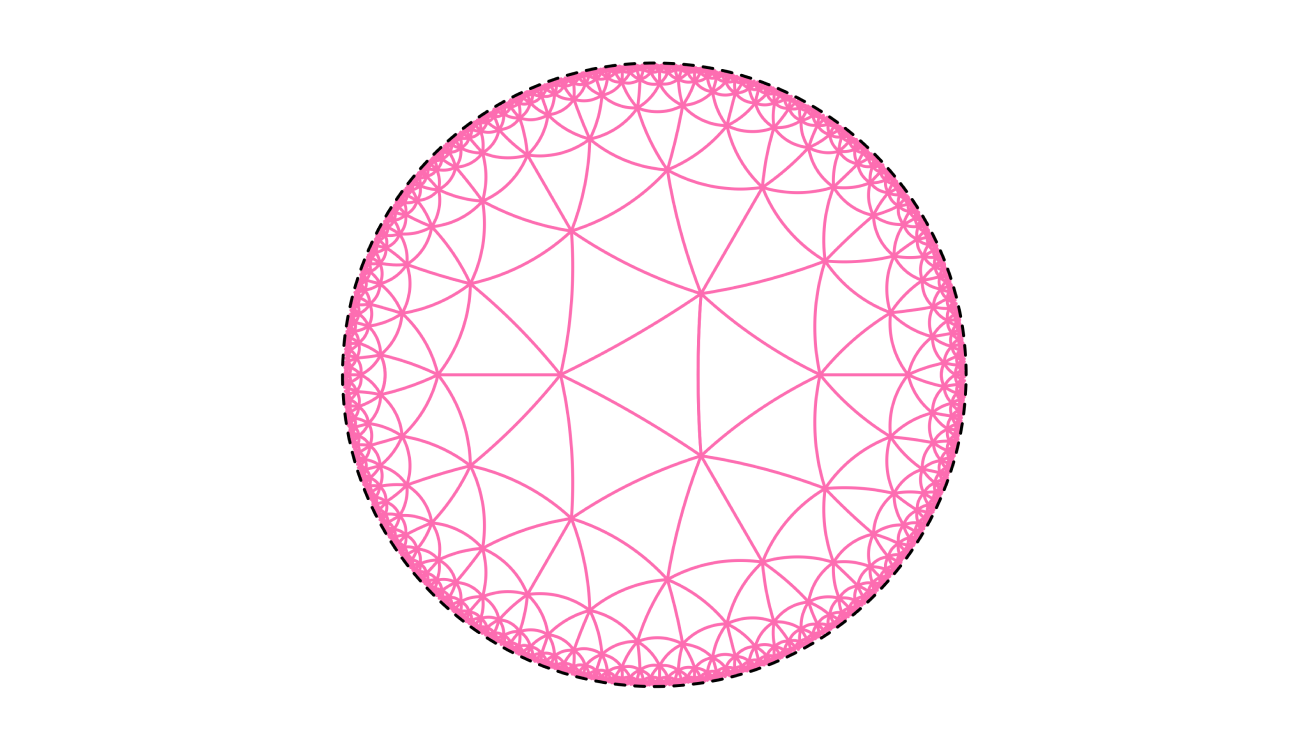# Visualizing Infinity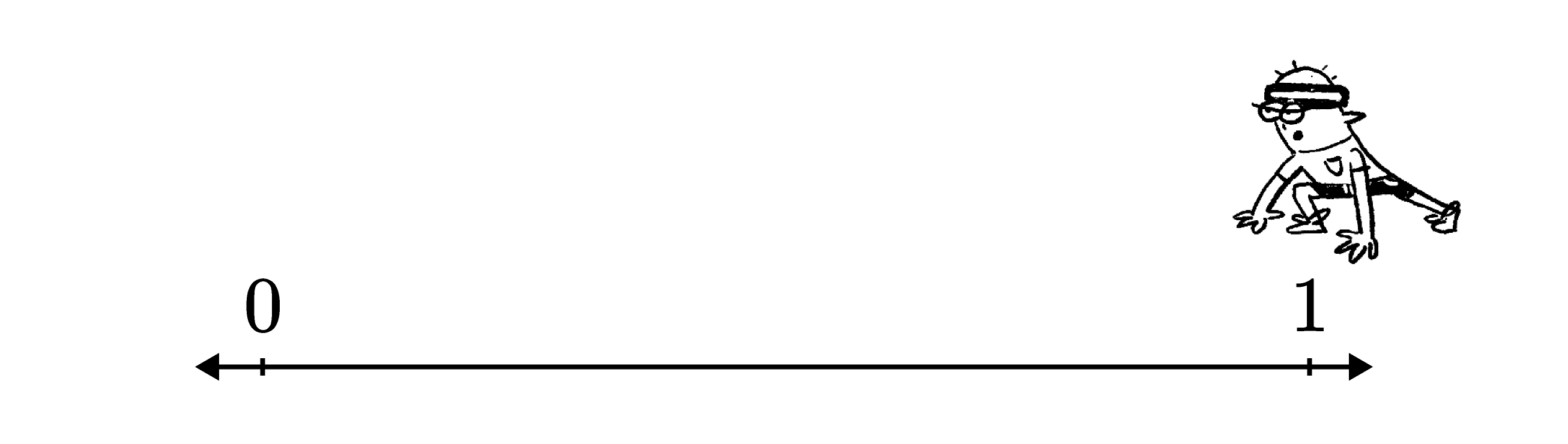Suppose this runner, Zeno, wants to start at the point marked "$1$" above and cross the vertical line at "$0$". However, because of the strange way that Zeno runs, each step he takes only brings him half of the remaining distance to $0.$

In order to reach $0,$ what would the value of $\text{Step}$ have to reach?

# Visualizing Infinity

Now let's visualize the series that describes Zeno's cumulative progress $\left(\frac{1}{2} + \frac{1}{4} + \frac{1}{8} + \frac{1}{16} + \cdots\right)$ geometrically: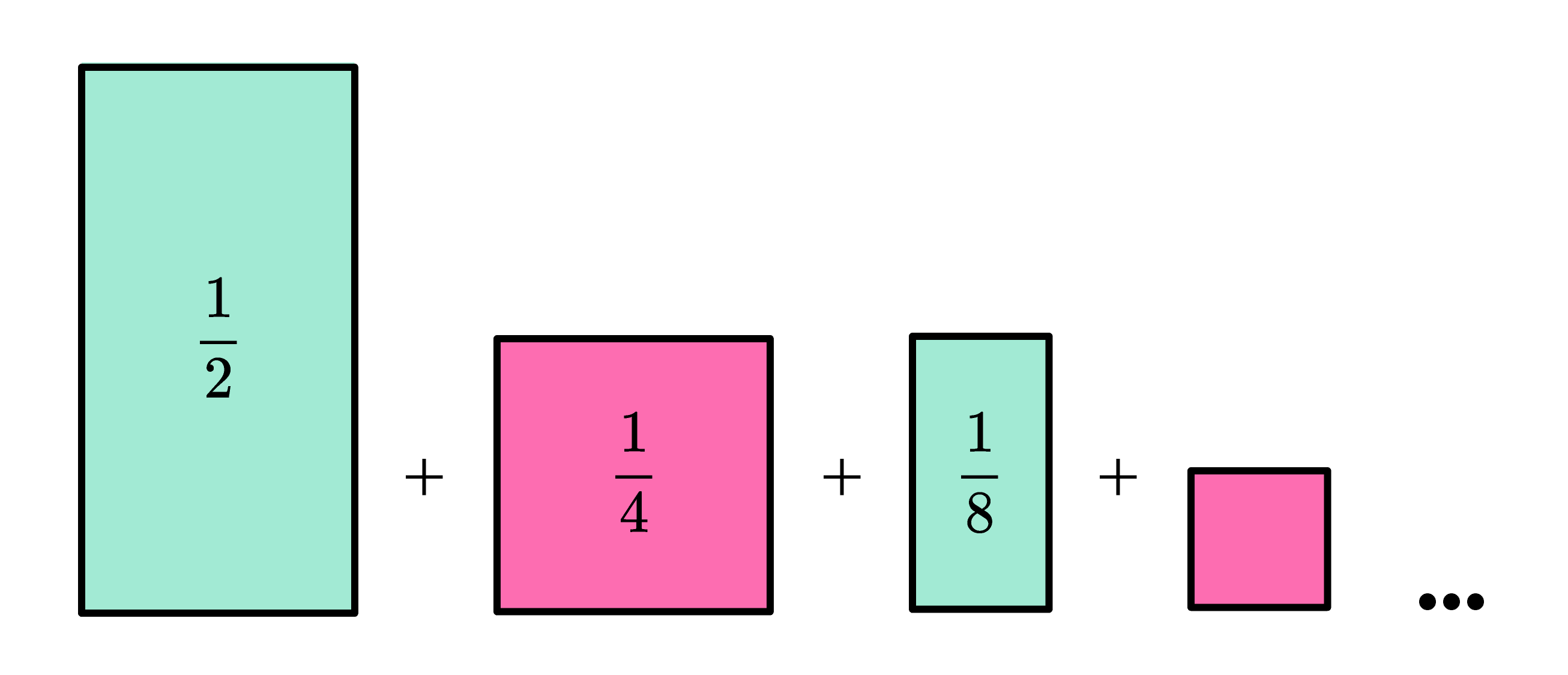All of the rectangles and squares in this sequence of shapes can pack within one unit square, just as all of Zeno's steps fit between $1$ and $0$ on the line that Zeno was running along: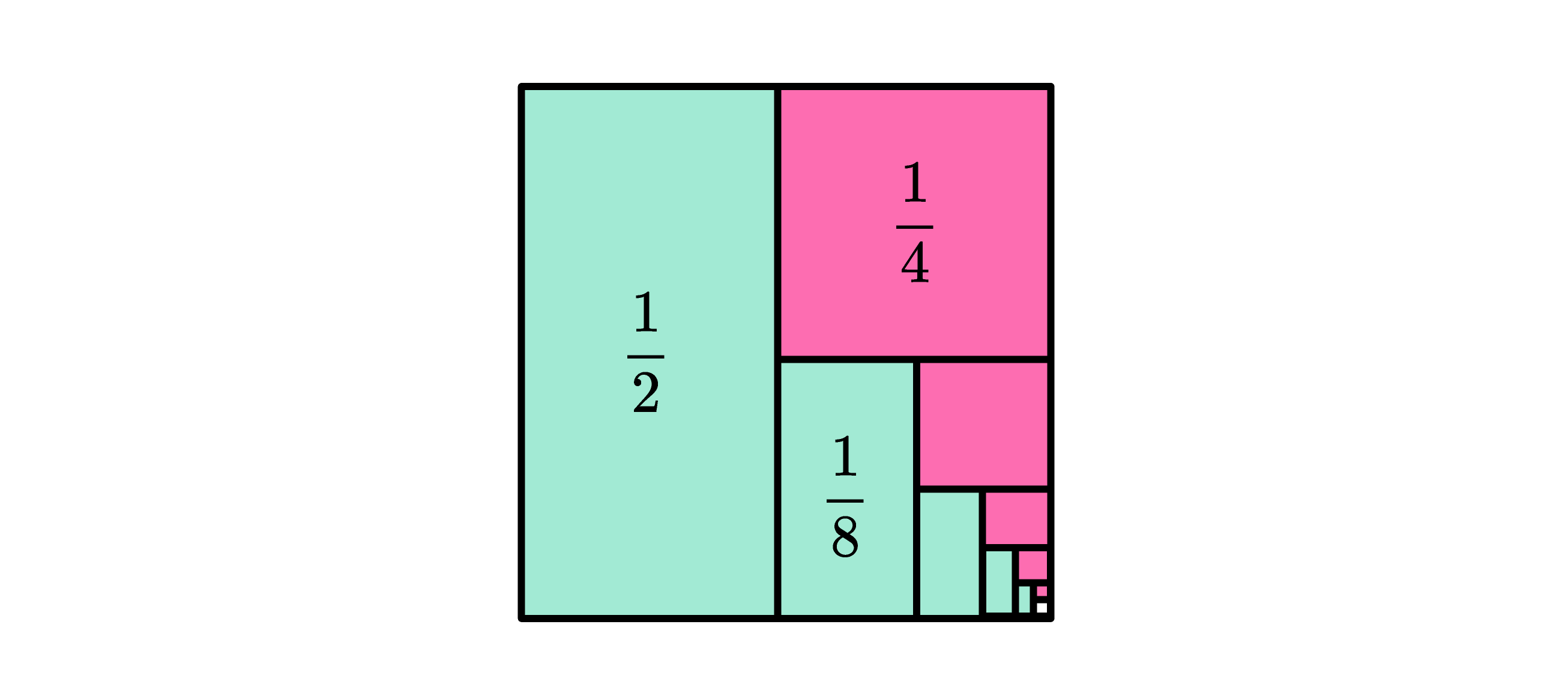# Visualizing Infinity

Here's another way to think about creating the figure below:

To start, a one-unit square is cut in half into two rectangles. The left rectangle is colored teal and the right rectangle is cut into two squares. Then the top of those two squares is colored pink and the bottom square is cut in half into two rectangles. This process is repeated over and over.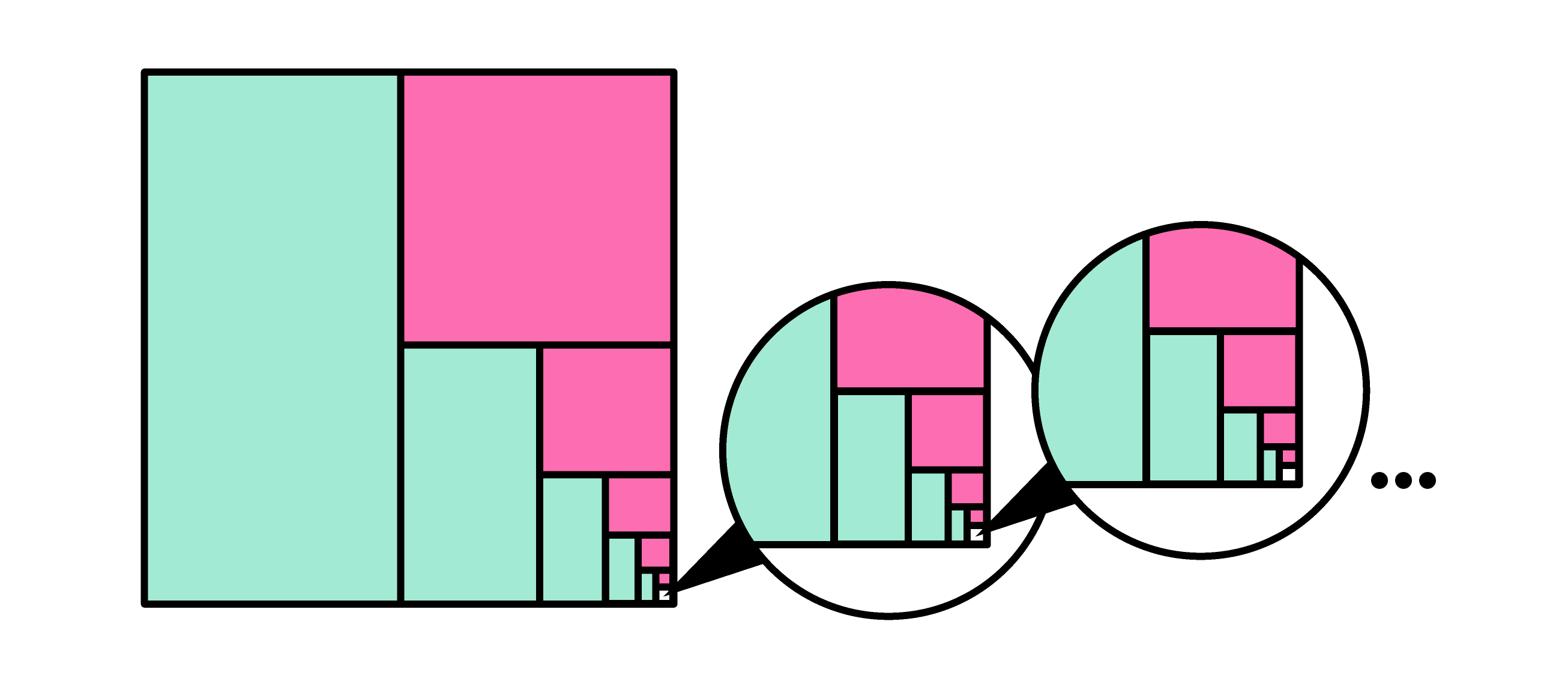What fraction of the original square's area is left entirely uncolored by this process?

# Visualizing Infinity

One strange observation about Zeno's journey is that his left and right feet aren't doing the same amount of legwork.

Let's say that Zeno's first step, the one that covers the first half of the distance, is one he makes with his left foot.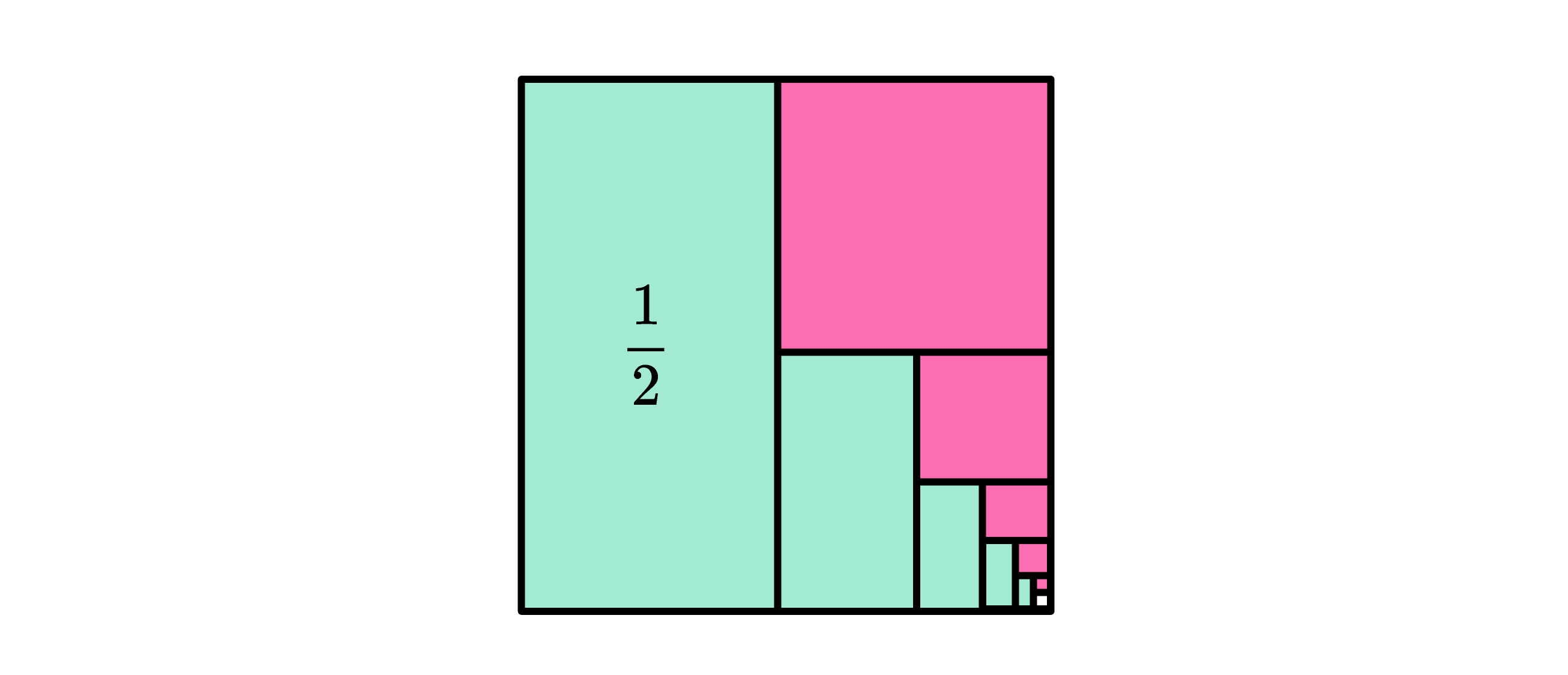If that's the case, then this follows:

The total pink area in this figure corresponds to the total distance of steps that Zeno takes with his right foot and the total green area corresponds to the total distance of steps Zeno takes with his left foot.

What fraction of the total distance from $1$ to $0$ is covered by steps Zeno takes with his right foot? (This is equivalent to the total pink area in the figure.)

# Visualizing Infinity

There's nothing stopping us from doing the same sort of thing with other shapes.

Start with an equilateral triangle that has an area of $1,$ and cut it into four congruent triangles, coloring three of them. If we repeat this process indefinitely, we will generate the spiral of triangles shown below, all of them equilateral: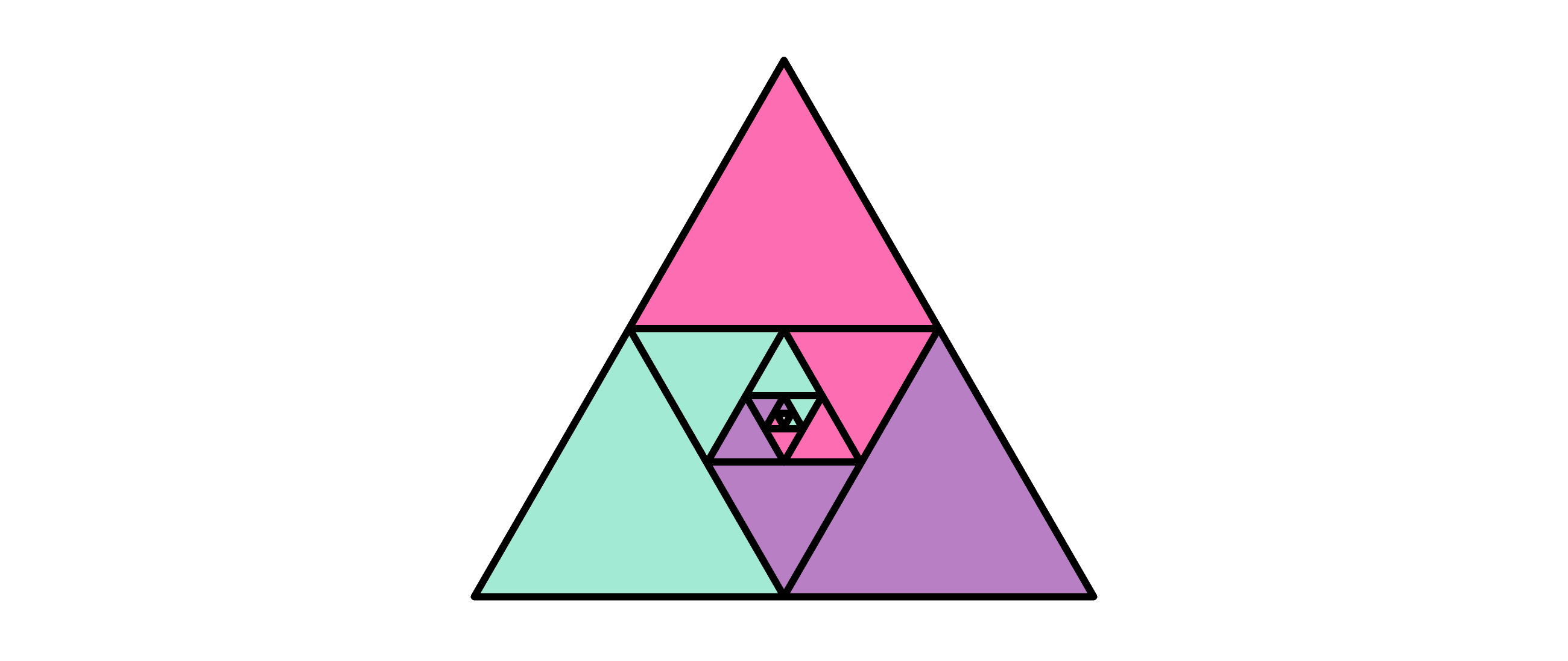Focusing on just one color (say, green), what does the above visualization show?

# Visualizing Infinity

Lastly, here's a quick demonstration of how this course will approach visualizing infinities that don't "fit" in two-dimensional Euclidean geometry.

The geometry that we'll use instead is called hyperbolic geometry. The images below show what it looks like to cover space with congruent equilateral triangles in "traditional" 2D, Euclidean space (shown on the left) and in hyperbolic space (shown on the right).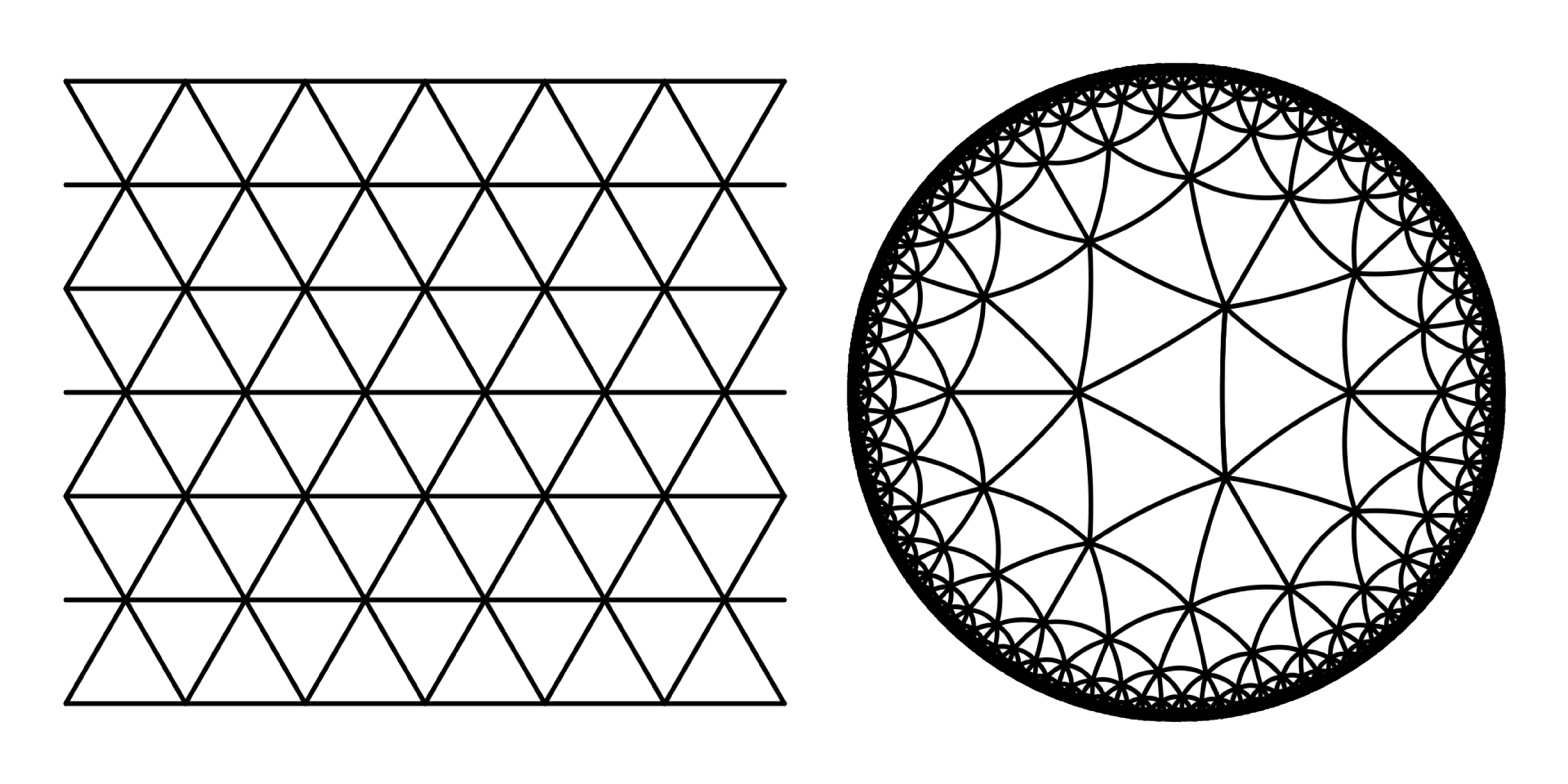In the Euclidean tessellation, six equilateral triangles meet together at each point of convergence. In the hyperbolic tessellation, seven equilateral triangles can all come together at one point.

Why is this what hyperbolic space looks like?

Later in this course, we'll go into more depth on the nature of hyperbolic geometry.

For now, you can think of the visualization of hyperbolic space shown above as a "fisheye" view of a universe we can barely imagine. Light bends as it comes through the window so that triangles that are infinitely far away can be seen at the edge of the window.

# Visualizing Infinity

If you tessellate with regular hexagons rather than triangles, what would the corresponding hyperbolic tessellation look like?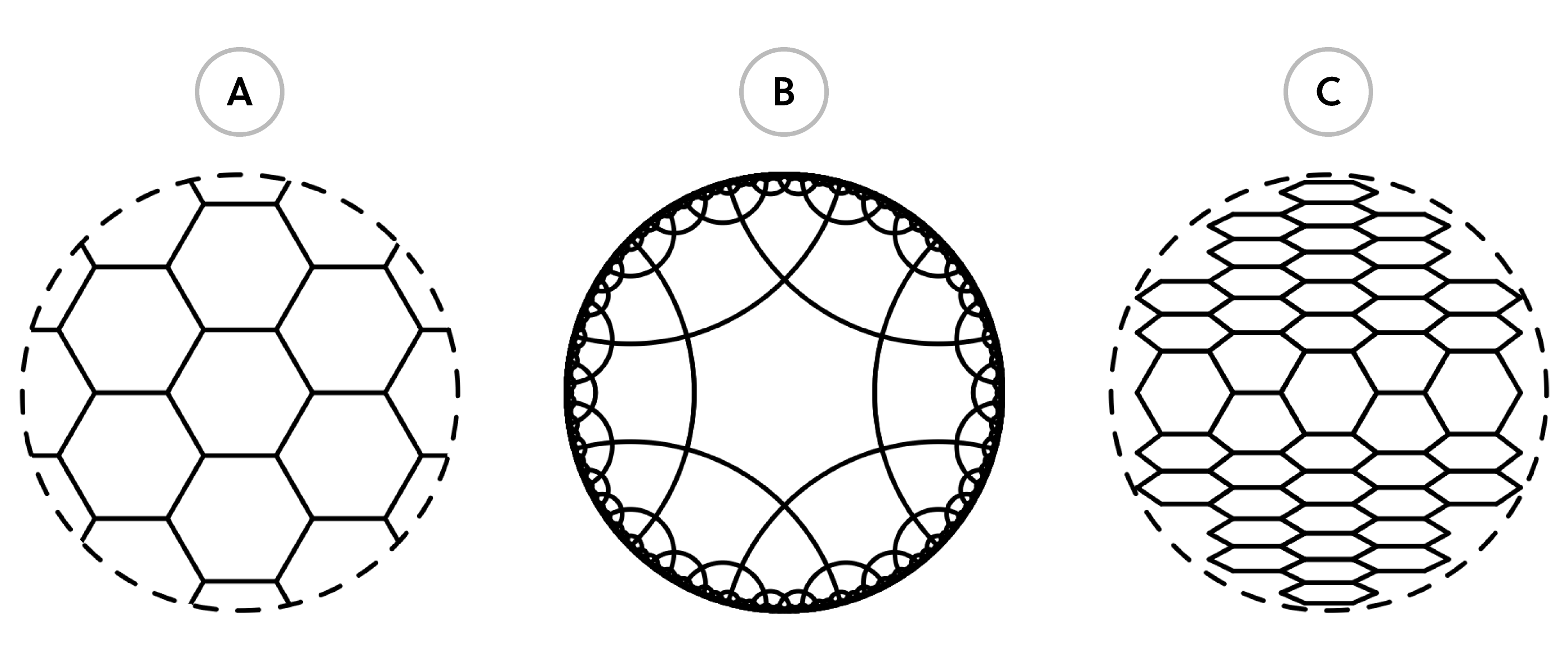# Visualizing Infinity

Hyperbolic geometry and infinite series are both topics this course will cover in much greater detail in future quizzes.

Overall, this course approaches introducing infinity in two ways:

1. The study of infinity as it is used in set theory, culminating in the derivation of Cantor's theorem, one of the deepest results in all of mathematics$\\[0.8em]$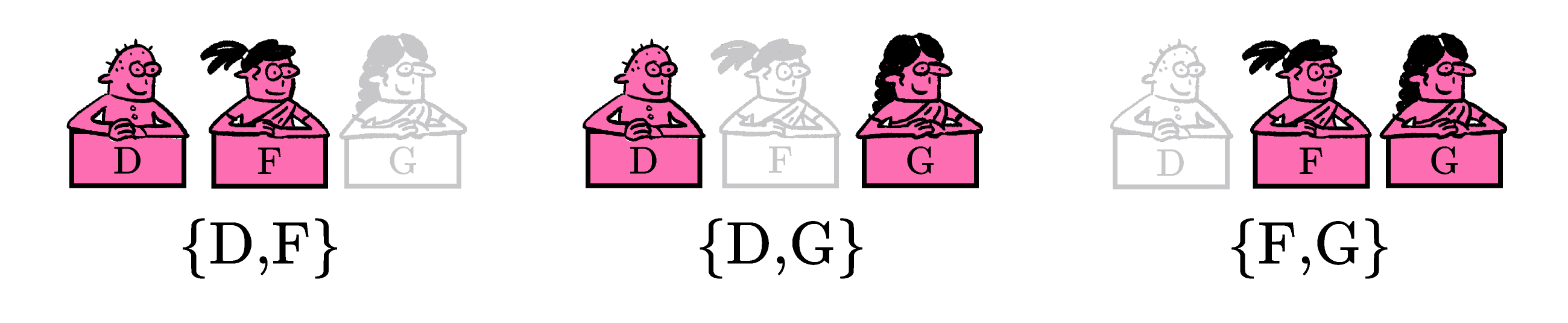2. Applying geometry to explore the continuous flavors of infinity found in geometry and calculus. This portion of the course content will cover limits, fractals, hyperbolic space, and other mind-warping mathematical ideas.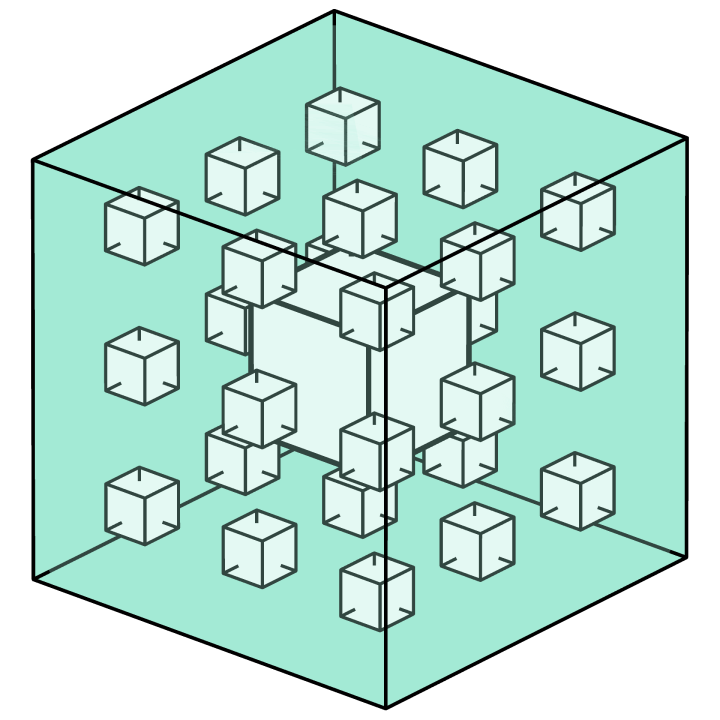In this course, you'll approach the limits of thought in a way only possible with mathematics!

# Visualizing Infinity

×